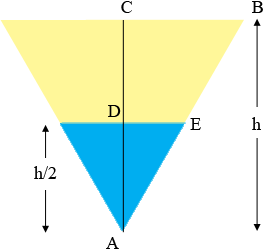SEARCH HOMEMath Central Quandaries & QueriesQuestion from Clarice, a student: A trough having an equilateral triangle end sections has sides equal to 0.4 m and 7m long. What is the volume of the liquid in the container if the depth of the water is one half the depth of the trough?Hi Clarice,

The volume of water in the trough is the area of the triangular cross section of the surface of the water at the end of the trough, times the length of the trough. I drew the triangular cross section of the end of the trough.The height of the trough is $h$ m so the height of the water in the trough is $h/2$ m. $C$ is the midpoint of the top of the trough. Triangles $ABC$ and $AED$ are similar.

Can you complete the problem?
PennyMath Central is supported by the University of Regina and the Imperial Oil Foundation.